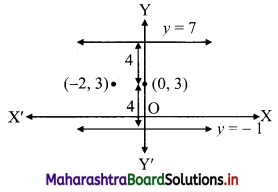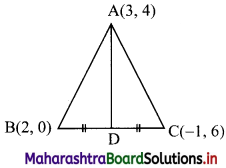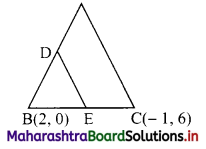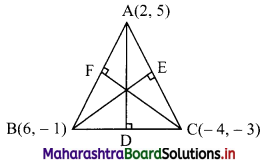# Maharashtra Board 11th Commerce Maths Solutions Chapter 5 Locus and Straight Line Ex 5.3

Balbharati Maharashtra State Board 11th Commerce Maths Solution Book Pdf Chapter 5 Locus and Straight Line Ex 5.3 Questions and Answers.

## Maharashtra State Board 11th Commerce Maths Solutions Chapter 5 Locus and Straight Line Ex 5.3

Question 1.
Write the equation of the line:
(a) parallel to the X-axis and at a distance of 5 units from it and above it.
(b) parallel to the Y-axis and at a distance of 5 units from it and to the left of it.
(c) parallel to the X-axis and at a distance of 4 units from the point (-2, 3).
Solution:
(a) Equation of a line parallel to the X-axis is y = k.
Since the line is at a distance of 5 units above the X-axis.
∴ k = 5
∴ the equation of the required line is y = 5.

(b) Equation of a line parallel to the Y-axis is x = h.
Since the line is at a distance of 5 units to the left of the Y-axis.
∴ h = -5
∴ the equation of the required line is x = -5.

(c) Equation of a line parallel to the X-axis is of the form y = k (k > 0 or k < 0).
Since, the line is at a distance of 4 units from the point (-2, 3).
∴ k = 3 + 4 = 7 or k = 3 – 4 = -1
∴ the equation of the required line is y = 7 or y = -1.Question 2.
Obtain the equation of the line:
(a) parallel to the X-axis and making an intercept of 3 units on the Y-axis.
(b) parallel to the Y-axis and making an intercept of 4 units on the X-axis.
Solution:
(a) Equation of a line parallel to X-axis with y-intercept ‘k’ is y = k.
Here, y-intercept = 3
∴ the equation of the required line is y = 3.

(b) Equation of a line parallel to Y-axis with x-intercept ‘h’ is x = h.
Here, x-intercept = 4
∴ the equation of the required line is x = 4.Question 3.
Obtain the equation of the line containing the point:
(a) A(2, -3) and parallel to the Y-axis.
(b) B(4, -3) and parallel to the X-axis.
Solution:
(a) Equation of a line parallel to the Y-axis is of the form x = h.
Since, the line passes through A(2, -3).
∴ h = 2
∴ the equation of the required line is x = 2.

(b) Equation of a line parallel to the X-axis is of the form y = k.
Since, the line passes through B(4, -3)
∴ k = -3
∴ the equation of the required line is y = -3.

Question 4.
Find the equation of the line passing through the points A(2, 0) and B(3, 4).
Solution:
The required line passes through the points A(2, 0) = (x1, y1) and B(3, 4) = (x2, y2) say.
Equation of the line in two-point form is
$$\frac{y-y_{1}}{y_{2}-y_{1}}=\frac{x-x_{1}}{x_{2}-x_{1}}$$
∴ the equation of the required line is
∴ $$\frac{y-0}{4-0}=\frac{x-2}{3-2}$$
∴ $$\frac{y}{4}=\frac{x-2}{1}$$
∴ y = 4(x – 2)
∴ y = 4x – 8
∴ 4x – y – 8 = 0

Check:
If the points A(2, 0) and B(3, 4) satisfy 4x – y – 8 = 0, then our answer is correct.
For point A(2, 0),
L.H.S. = 4x – y – 8
= 4(2) – 0 – 8
= 8 – 8
= 0
= R.H.S.
For point B(3, 4),
L.H.S. = 4x – y – 8
= 4(3) – 4 – 8
= 12 – 12
= 0
= R.H.S.Question 5.
Line y = mx + c passes through the points A(2, 1) and B(3, 2). Determine m and c.
Solution:
Given, A(2, 1) and B(3, 2).
Equation of a line in two-point form is
$$\frac{y-y_{1}}{y_{2}-y_{1}}=\frac{x-x_{1}}{x_{2}-x_{1}}$$
∴ the equation of the passing through A and B line is
∴ $$\frac{y-1}{2-1}=\frac{x-2}{3-2}$$
∴ $$\frac{y-1}{1}=\frac{x-2}{1}$$
∴ y – 1 = x – 2
∴ y = x – 1
Comparing this equation with y = mx + c, we get
m = 1 and c = -1

Alternate method:
Points A(2, 1) and B(3, 2) lie on the line y = mx + c.
∴ They must satisfy the equation.
∴ 2m + c = 1 ……..(i)
and 3m + c = 2 ……(ii)
equation (ii) – equation (i) gives m = 1
Substituting m = 1 in (i), we get
2(1) – c = 1
∴ c = 1 – 2 = -1

Question 6.
The vertices of a triangle are A(3, 4), B(2, 0), and C(-1, 6). Find the equations of
(a) side BC
(c) the midpoints of sides AB and BC.
Solution:
Vertices of ∆ABC are A(3, 4), B(2, 0) and C(-1, 6).
(a) Equation of a line in two-point form is
$$\frac{y-y_{1}}{y_{2}-y_{1}}=\frac{x-x_{1}}{x_{2}-x_{1}}$$
∴ the equation of the side BC is
$$\frac{y-0}{6-0}=\frac{x-2}{-1-2}$$ ……[B = (x1, y1) = (2, 0), C = (x2, y2) = (-1, 6)]
∴ $$\frac{y}{6}=\frac{x-2}{-3}$$
∴ y = -2(x – 2)
∴ 2x + y – 4 = 0

(b) Let D be the midpoint of side BC.
Then, AD is the median through A.
∴ D = $$\left(\frac{2-1}{2}, \frac{0+6}{2}\right)=\left(\frac{1}{2}, 3\right)$$
The median AD passes through the points A(3, 4) and D($$\frac{1}{2}$$, 3)∴ the equation of the median AD is
$$\frac{y-4}{3-4}=\frac{x-3}{\frac{1}{2}-3}$$
∴ $$\frac{y-4}{-1}=\frac{x-3}{-\frac{5}{2}}$$
∴ $$\frac{1}{2}$$ (y – 4) = x – 3
∴ 5y – 20 = 2x – 6
∴ 2x – 5y + 14 = 0

(c) Let D and E be the midpoints of side AB and side BC respectively.
∴ D = $$\left(\frac{3+2}{2}, \frac{4+0}{2}\right)=\left(\frac{5}{2}, 2\right)$$ and
E = $$\left(\frac{2-1}{2}, \frac{0+6}{2}\right)=\left(\frac{1}{2}, 3\right)$$the equation of the line DE is
$$\frac{y-2}{3-2}=\frac{x-\frac{5}{2}}{\frac{1}{2}-\frac{5}{2}}$$
∴ $$\frac{y-2}{1}=\frac{2 x-5}{-4}$$
∴ -4(y – 2) = 2x – 5
∴ -4y + 8 = 2x – 5
∴ 2x + 4y – 13 = 0Question 7.
Find the x and y-intercepts of the following lines:
(a) $$\frac{x}{3}+\frac{y}{2}=1$$
(b) $$\frac{3 x}{2}+\frac{2 y}{3}=1$$
(c) 2x – 3y + 12 = 0
Solution:
(a) Given equation of the line is $$\frac{x}{3}+\frac{y}{2}=1$$
This is of the form $$\frac{x}{a}+\frac{y}{b}=1$$,
where x-intercept = a, y-intercept = b
∴ x-intercept = 3, y-intercept = 2

(b) Given equation of the line is $$\frac{3 x}{2}+\frac{2 y}{3}=1$$
∴ $$\frac{x}{\left(\frac{2}{3}\right)}+\frac{y}{\left(\frac{3}{2}\right)}=1$$
This is of the form $$\frac{x}{a}+\frac{y}{b}=1$$,
where x-intercept = a, y-intercept = b
∴ x-intercept = $$\frac{2}{3}$$ and y-intercept = $$\frac{3}{2}$$

(c) Given equation of the line is 2x – 3y + 12 = 0
∴ 2x – 3y = -12
∴ $$\frac{2 x}{(-12)}-\frac{3 y}{(-12)}=1$$
∴ $$\frac{x}{-6}+\frac{y}{4}=1$$
This is of the form $$\frac{x}{a}+\frac{y}{b}=1$$,
where x-intercept = a, y-intercept = b
∴ x-intercept = -6 and y-intercept = 4

Question 8.
Find the equations of a line containing the point A(3, 4) and make equal intercepts on the co-ordinate axes.
Solution:
Let the equation of the line be
$$\frac{x}{a}+\frac{y}{b}=1$$ …..(i)
Since, the required line make equal intercepts on the co-ordinate axes.
∴ a = b
∴ (i) reduces to x + y = a …..(ii)
Since the line passes through A(3, 4).
∴ 3 + 4 = a
i.e. a = 7
Substituting a = 7 in (ii) to get
x + y = 7Question 9.
Find the equations of the altitudes of the triangle whose vertices are A(2, 5), B(6, -1) and C(-4, -3).
Solution:A(2, 5), B(6, -1), C(-4, -3) are the vertices of ∆ABC.
Let AD, BE and CF be the altitudes through the vertices A, B and C respectively of ∆ABC.
Slope of BC = $$\frac{-3-(-1)}{-4-6}=\frac{-2}{-10}=\frac{1}{5}$$
Since, altitude AD passes through (2, 5) and has slope -5.
∴ the equation of the altitude AD is
y – 5 = -5(x – 2)
∴ y – 5 = – 5x + 10
∴ 5x + y – 15 = 0
Now, slope of AC = $$\frac{-3-5}{-4-2}=\frac{-8}{-6}=\frac{4}{3}$$
∴ slope of BE = $$\frac{-3}{4}$$ …..[∵ BE ⊥ AC]
Since, altitude BE passes through (6, -1) and has slope $$\frac{-3}{4}$$.
∴ the equation of the altitude BE is
y – (-1) = $$\frac{-3}{4}$$(x – 6)
∴ 4(y + 1) = -3(x – 6)
∴ 3x + 4y – 14 = 0
Also, slope of AB = $$\frac{-1-5}{6-2}=\frac{-6}{4}=\frac{-3}{2}$$
∴ slope of CF = $$\frac{2}{3}$$ ………[∵ CF ⊥ AB]
Since, altitude CF passes through (-4, -3) and has slope $$\frac{2}{3}$$.
∴ the equation of the altitude CF is
y – (-3) = $$\frac{2}{3}$$ [x – (-4)]
∴ 3(y + 3) = 2(x + 4)
∴ 2x – 3y – 1 = 0